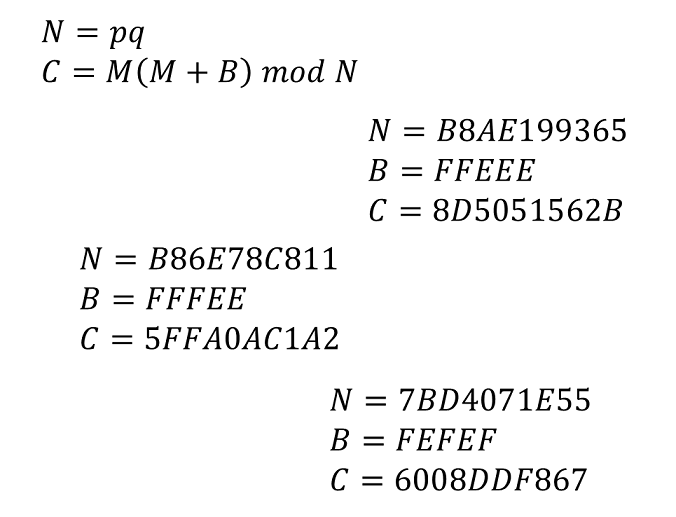For this challenge we have a binary that encrypts data and an encrypted file.

After some reversing we understood that the binary is only generating randomness using rand() and XORing it wirh each byte of the content. As the seed is the timestamp in seconds and we can get the creation date of the encrypted file this first step if the challenge was trivial.

Just compile this source and run it:

`\$ ./solver ecrypt1.bin output.png 1416667590`

We get this image back:Here’s the real challenge :) of course we need to decrypt the three different C. N is not that big, however it’s still to large for bruteforcing. Luckily we can reduce the bruteforcing space by factorizing N and finding an M such that these equations are satisfied:

• C mod p == M(M+B) mod p

• C mod q == M(M+B) mod q

We will find possible candidates for (M mod p) and (M mod q) that we can combine back together using the Chinese Reminder Theorem to obtain (M mod N). This script automates the process.

flag: SECCON{Ra_b1_N}

fox